# Lie group

In mathematics, a Lie group (pronounced /liː/ LEE) is a group that is also a differentiable manifold. A manifold is a space that locally resembles Euclidean space, whereas groups define the abstract concept of a binary operation along with the additional properties it must have to be a group, for instance multiplication and the taking of inverses (division), or equivalently, the concept of addition and the taking of inverses (subtraction). Combining these two ideas, one obtains a continuous group where multiplying points and their inverses are continuous. If the multiplication and taking of inverses are smooth (differentiable) as well, one obtains a Lie group. Lie groups provide a natural model for the concept of continuous symmetry, a celebrated example of which is the rotational symmetry in three dimensions (given by the special orthogonal group ). Lie groups are widely used in many parts of modern mathematics and physics. Lie groups were first found by studying matrix subgroups contained in or , the groups of invertible matrices over or . These are now called the classical groups, as the concept has been extended far beyond these origins. Lie groups are named after Norwegian mathematician Sophus Lie (1842–1899), who laid the foundations of the theory of continuous transformation groups. Lie's original motivation for introducing Lie groups was to model the continuous symmetries of differential equations, in much the same way that finite groups are used in Galois theory to model the discrete symmetries of algebraic equations. (Wikipedia).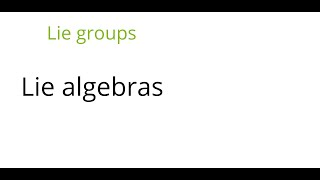Lie groups: Lie algebras

This lecture is part of an online graduate course on Lie groups. We define the Lie algebra of a Lie group in two ways, and show that it satisfied the Jacobi identity. The we calculate the Lie algebras of a few Lie groups. For the other lectures in the course see https://www.youtube.co

From playlist Lie groups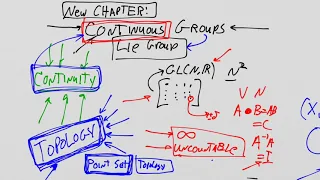Lie Groups and Lie Algebras: Lesson 13 - Continuous Groups defined

Lie Groups and Lie Algebras: Lesson 13 - Continuous Groups defined In this lecture we define a "continuous groups" and show the connection between the algebraic properties of a group with topological properties. Please consider supporting this channel via Patreon: https://www.patreon.co

From playlist Lie Groups and Lie Algebras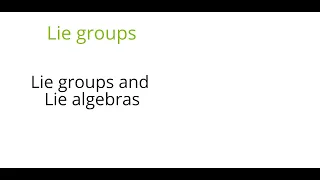Lie groups: Lie groups and Lie algebras

This lecture is part of an online graduate course on Lie groups. We discuss the relation between Lie groups and Lie algebras, and give several examples showing how they behave differently. Lie algebras turn out to correspond more closely to the simply connected Lie groups. We then explain

From playlist Lie groups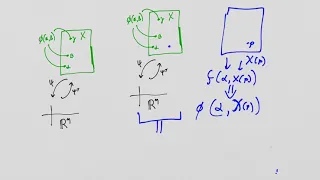Lie Groups and Lie Algebras: Lesson 22 - Lie Group Generators

Lie Groups and Lie Algebras: Lesson 22 - Lie Group Generators A Lie group can always be considered as a group of transformations because any group can transform itself! In this lecture we replace the "geometric space" with the Lie group itself to create a new collection of generators. P

From playlist Lie Groups and Lie Algebras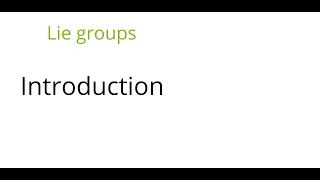Lie groups: Introduction

This lecture is part of an online graduate course on Lie groups. We give an introductory survey of Lie groups theory by describing some examples of Lie groups in low dimensions. Some recommended books: Lie algebras and Lie groups by Serre (anything by Serre is well worth reading) Repre

From playlist Lie groups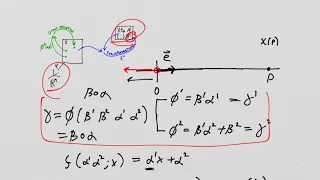Lie Groups and Lie Algebras: Lesson 16 - representations, connectedness, definition of Lie Group

Lie Groups and Lie Algebras: Lesson 16 - representations, connectedness, definition of Lie Group We cover a few concepts in this lecture: 1) we introduce the idea of a matrix representation using our super-simple example of a continuous group, 2) we discuss "connectedness" and explain tha

From playlist Lie Groups and Lie Algebras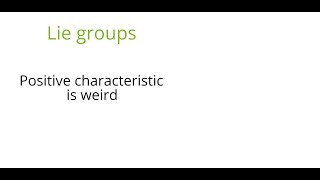Lie groups: Positive characteristic is weird

This lecture is part of an online graduate course on Lie groups. We give several examples to show that, over fields of positive characteristic, Lie algebras can behave strangely, and have a weaker connection to Lie groups. In particular the Lie algebra does not generate the ring of all in

From playlist Lie groups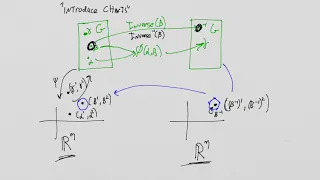Lie Groups and Lie Algebras: Lesson 26: Review!

Lie Groups and Lie Algebras: Lesson 26: Review! It never hurts to recap! https://www.patreon.com/XYLYXYLYX

From playlist Lie Groups and Lie Algebras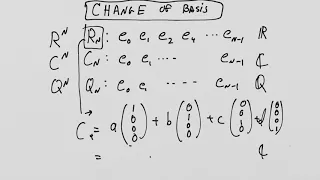Lie Groups and Lie Algebras: Lesson 3 - Classical Groups Part I

Lie Groups and Lie Algebras: Lesson 3 - Classical Groups Part I We introduce the idea of the classical matrix groups and their associated carrier spaces. Please consider supporting this channel via Patreon: https://www.patreon.com/XYLYXYLYX

From playlist Lie Groups and Lie Algebras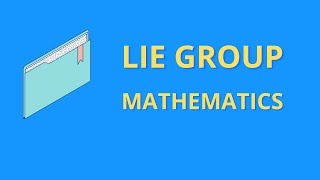Lie Groups for Deep Learning w/ Graph Neural Networks

Lie Groups encode the symmetry of systems. We examine actions of a Lie group on a vector space, given their algebraic, topological and analysis based connectome. Deep Learning algorithms for Graph Neural Networks (GNN) are non trivial, and to understand them Lie Groups are essential! A r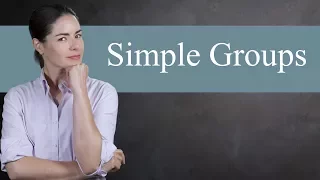Simple Groups - Abstract Algebra

Simple groups are the building blocks of finite groups. After decades of hard work, mathematicians have finally classified all finite simple groups. Today we talk about why simple groups are so important, and then cover the four main classes of simple groups: cyclic groups of prime order

From playlist Abstract Algebra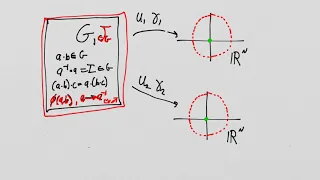Lie Groups and Lie Algebras: Lesson 38 - Preparation for the concept of a Universal Covering Group

Lie Groups and Lie Algebras: Lesson 38 - Preparation for the Universal Covering Group concept In this lesson we examine another amazing connection between the algebraic properties of the Lie groups with topological properties. We will lay the foundation to understand how discrete invaria

From playlist Lie Groups and Lie Algebras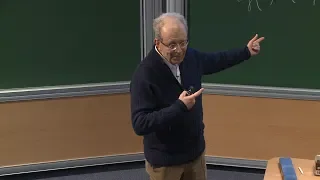Jean Michel BISMUT - Fokker-Planck Operators and the Center of the Enveloping Algebra

The heat equation method in index theory gives an explicit local formula for the index of a Dirac operator. Its Lagrangian counterpart involves supersymmetric path integrals. Similar methods can be developed to give a geometric formula for semi simple orbital integrals associated with the

From playlist Integrability, Anomalies and Quantum Field TheoryMacro Activity- The FEDexes Fix the the Economy

This is an awesome activity that will help you apply what you are learning in your macroeconomics class. Specifically, monetray policy and the role of the Federal Reserve. Teachers: I made a video that gives you more details about how to run this activity and gives you the link to the the

From playlist Clifford's Favorite Videos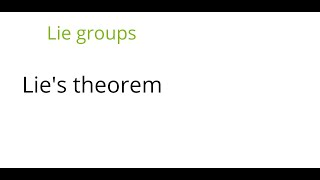Lie groups: Lie's theorem

This lecture is part of an online graduate course on Lie groups. This lecture is about Lie's theorem, which implies that a complex solvable Lie algebra is isomorphic to a subalgebra of the upper triangular matrices. . For the other lectures in the course see https://www.youtube.com/playl

From playlist Lie groups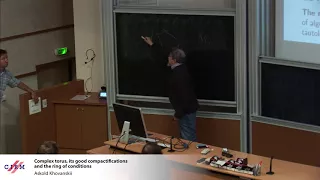Askold Khovanskii: Complex torus, its good compactifications and the ring of conditions

Abstract: Let X be an algebraic subvariety in (ℂ∗)n. According to the good compactifification theorem there is a complete toric variety M⊃(ℂ∗)n such that the closure of X in M does not intersect orbits in M of codimension bigger than dimℂX. All proofs of this theorem I met in literature ar

From playlist Algebraic and Complex Geometry